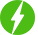# Master Complete Statistics For Computer Science – I

## Requirements

• Knowledge of Applied Probability
• Knowledge of Calculus

## Description

In today’s engineering curriculum, topics on probability and statistics play a major role, as the statistical methods are very helpful in analyzing the data and interpreting the results.

When an aspiring engineering student takes up a project or research work, statistical methods become very handy.

Hence, the use of a well-structured course on probability and statistics in the curriculum will help students understand the concept in depth, in addition to preparing for examinations such as for regular courses or entry-level exams for postgraduate courses.

In order to cater the needs of the engineering students, content of this course, are well designed. In this course, all the sections are well organized and presented in an order as the contents progress from basics to higher level of statistics.

As a result, this course is, in fact, student friendly, as I have tried to explain all the concepts with suitable examples before solving problems.

This 150+ lecture course includes video explanations of everything from Random Variables, Probability Distribution, Statistical Averages, Correlation, Regression, Characteristic Function, Moment Generating Function and Bounds on Probability, and it includes more than 90+ examples (with detailed solutions) to help you test your understanding along the way. “Master Complete Statistics For Computer Science – I” is organized into the following sections:

• Introduction
• Discrete Random Variables
• Continuous Random Variables
• Cumulative Distribution Function
• Special Distribution
• Two – Dimensional Random Variables
• Random Vectors
• Function of One Random Variable
• One Function of Two Random Variables
• Two Functions of Two Random Variables
• Measures of Central Tendency
• Mathematical Expectations and Moments
• Measures of Dispersion
• Skewness and Kurtosis
• Statistical Averages – Solved Examples
• Expected Values of a Two-Dimensional Random Variables
• Linear Correlation
• Correlation Coefficient
• Properties of Correlation Coefficient
• Rank Correlation Coefficient
• Linear Regression
• Equations of the Lines of Regression
• Standard Error of Estimate of Y on X and of X on Y
• Characteristic Function and Moment Generating Function
• Bounds on Probabilities

## Who this course is for:

• Current Probability and Statistics students
• Students of Machine Learning, Artificial Intelligence, Data Science, Computer Science, Electrical Engineering , as Statistics is the prerequisite course to Machine Learning, Data Science, Computer Science and Electrical Engineering
• Anyone who wants to study Statistics for fun after being away from school for a while.#### WebHelperApp

Rely on the Coupon WebHelperApp team's decade of expertise in sourcing 100% off Udemy coupons. Our commitment includes rigorous verification and daily updates to ensure a reliable assortment of fully functional coupon codes. We specialize in promptly uncovering fresh offers, often with limited availability, necessitating swift action on your part.

We will be happy to hear your thoughts